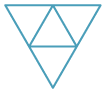#### Question 1

Fill in the blanks to describe the attributes of each pyramid.

a A square-based pyramid has How many faces? faces, How many edges? edges and How many vertices? vertices. A pentagonal-based pyramid has How many faces? faces, How many edges? edges and How many vertices? vertices.

#### Question 2

Match the net to the pyramid.

ABCa b c The net for a triangular-based pyramid is net: A B C The net for a hexagonal-based pyramid is net: A B C The net for an square-based pyramid is net: A B C